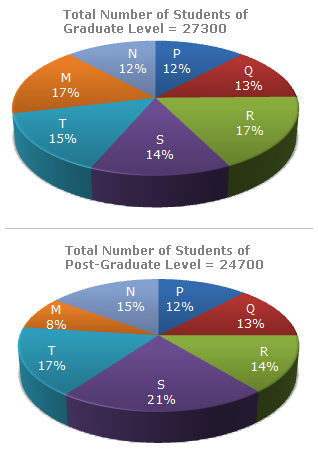# Data Interpretation - Pie Charts - Discussion

The following pie-charts show the distribution of students of graduate and post-graduate levels in seven different institutes in a town.5.

Total number of students studying at post-graduate level from institutes N and P is

 [A]. 5601 [B]. 5944 [C]. 6669 [D]. 8372

Explanation:

 Required number = (15% of 24700) + (12% of 24700) = 3705 + 2964 = 6669.